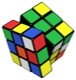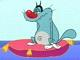# Math Is Fun Forum

Discussion about math, puzzles, games and fun.   Useful symbols: ÷ × ½ √ ∞ ≠ ≤ ≥ ≈ ⇒ ± ∈ Δ θ ∴ ∑ ∫  π  -¹ ² ³ °

You are not logged in.

## #1 2009-08-10 00:41:12

mathew2
Guest

### prove it!

If a,b,c r are the lengths of a triangle whose area is S , then show that

4S*3^(1/2)

## #2 2009-08-10 04:41:25

juriguen
MemberRegistered: 2009-07-05
Posts: 59

### Re: prove it!

Hi!

I think I have found the solution based on this website:
http://planetmath.org/encyclopedia/BoundOnAreaOfRightTriangle.html

Lets go:

According to Heron's formula (http://en.wikipedia.org/wiki/Heron%27s_formula)

So, we are going to try to maximize the are of the triangle, subject to k = a^2 + b^2 + c^2 being constant, using Lagrange multipliers.

It is easier to work with S^2, and maximize it, so we set:

I felt lazy to solve the system of equations myself, so I plugged it into Mathematica (http://www.quickmath.com/webMathematica3/quickmath/page.jsp?s1=equations&s2=solve&s3=advanced).

The only valid solution with all a, b, c > 0 yields:

Therefore, and since we were trying to maximize S, we have:

Hope it helps!Jose

Make everything as simple as possible, but not simpler. -- Albert Einstein

Offline

## #3 2009-08-10 12:25:21

Kurre
MemberRegistered: 2006-07-18
Posts: 280

### Re: prove it!

We first use the cosine theorem on each of the squared sides:

Thus we get:

solving for
and using the areaforumla (
etc) :

To minimize this sum we can for example use jensens inequality.
http://en.wikipedia.org/wiki/Jensen%27s_inequality
We first assume that the triangle is acute, so all angles are less than
. Then
is convex, since
and
. Thus Jensens inequality applies and we get:

so

What if one angle is obtuse?
Then we can argue as follows. WLOG we assume

. Then there exists a corresponding triangle with sides a',b',c' and
and b=b' and c=c'. This triangle must have the same area since
but a'<a since it corresponds to a smaller angle. the new triangle is acute, so we can apply the result there, thus

Q.e.d

Offline